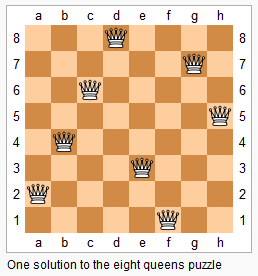# LeetCode: 51. N皇后¶

## 1、题目描述¶

n 皇后问题研究的是如何将 n 个皇后放置在 n×n 的棋盘上，并且使皇后彼此之间不能相互攻击。输入: 4

[".Q..",  // 解法 1
"...Q",
"Q...",
"..Q."],

["..Q.",  // 解法 2
"Q...",
"...Q",
".Q.."]
]



## 2、解题思路¶

• 回溯法
• 皇后问题的关键在于如何快速判断是否在攻击范围中

di = (r * n + c) % (n+1) + (0 if c >= r else n)


bdi = r + c

class Solution:
def solveNQueens(self, n: int) -> List[List[str]]:
lines = []

for i in range(n):
lines.append(['.'] * i + ["Q"] + ["."] * (n - i - 1))

res = []

row = set()
col = set()
diagonal = set()
back_diagonal = set()

temp = []

def dfs(r):
nonlocal row, col, diagonal, back_diagonal, n
if r == n:
res.append(["".join(x) for x in temp])
return
for c in range(n):
if r in row or c in col:
continue
# 对角线判断
# base =
di = (r * n + c) % (n+1) + (0 if c >= r else n)
# 反对角线判断
bdi = r + c

if di in diagonal or bdi in back_diagonal:
continue